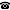# 2011 seminar talk: Non-absoluteness of model existence for infinitary logic

Talk held by Martin Koerwien (KGRC) at the KGRC seminar on 2011-10-06.

### Abstract

We discuss how much the notion '$$\sigma$$ has a model of size $$\aleph_\alpha$$' (where $$\sigma$$ is a sentence of $$L_{\omega_1,\omega}$$) can depend on set theoretic properties. After some general remarks and examples, we will focus on the presentation of a complete sentence for which model-existence in $$\aleph_3$$ is non-absolute modulo ZFC+GCH.

Kurt Gödel Research Center for Mathematical Logic. Währinger Straße 25, 1090 Wien, Austria.+43-1-4277-50501. Last updated: 2010-12-16, 04:37.